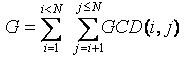favorite We need a little bit of your help to keep things running, click on this banner to learn more
Problems

# GCD Extreme

Given the value of n, you have to find the value of G, whereHere GCD(i, j) means the greatest common divisor of integer i and integer j.

For those who have trouble understanding summation notation, the meaning of G is given in the following code:

 G=0;for(i=1; i < n;i++)for(j=i+1;j<=n;j++){ G+=GCD(i,j);}/*Here GCD() is a function that finds the greatest common divisor of the two input numbers*/

Input

The input file contains at most 20000 lines of inputs. Each line contains an integer n (1 < n < 200001). The last line contains n = 0 and is not processed.

Output

For each line of input produce one line of output. This line contains the value of G for the corresponding n. The value of G will fit in a 64-bit signed integer.

Time limit 2 seconds
Memory limit 64 MiB
Input example #1
```10
100
20000
0
```
Output example #1
```67
13015
1153104356
```